$\newcommand{\NN}{\mathbb{N}} \newcommand{\CC}{\mathbb{C}} \newcommand{\GG}{\mathbb{G}} \newcommand{\LL}{\mathbb{L}} \newcommand{\PP}{\mathbb{P}} \newcommand{\QQ}{\mathbb{Q}} \newcommand{\RR}{\mathbb{R}} \newcommand{\VV}{\mathbb{V}} \newcommand{\ZZ}{\mathbb{Z}} \newcommand{\FF}{\mathbb{F}} \newcommand{\KK}{\mathbb{K}} \newcommand{\UU}{\mathbb{U}} \newcommand{\EE}{\mathbb{E}} \newcommand{\Aa}{\mathcal{A}} \newcommand{\Bb}{\mathcal{B}} \newcommand{\Cc}{\mathcal{C}} \newcommand{\Dd}{\mathcal{D}} \newcommand{\Ee}{\mathcal{E}} \newcommand{\Ff}{\mathcal{F}} \newcommand{\Gg}{\mathcal{G}} \newcommand{\Hh}{\mathcal{H}} \newcommand{\Ii}{\mathcal{I}} \newcommand{\Jj}{\mathcal{J}} \newcommand{\Kk}{\mathcal{K}} \newcommand{\Ll}{\mathcal{L}} \newcommand{\Mm}{\mathcal{M}} \newcommand{\Nn}{\mathcal{N}} \newcommand{\Oo}{\mathcal{O}} \newcommand{\Pp}{\mathcal{P}} \newcommand{\Qq}{\mathcal{Q}} \newcommand{\Rr}{\mathcal{R}} \newcommand{\Ss}{\mathcal{S}} \newcommand{\Tt}{\mathcal{T}} \newcommand{\Uu}{\mathcal{U}} \newcommand{\Vv}{\mathcal{V}} \newcommand{\Ww}{\mathcal{W}} \newcommand{\Xx}{\mathcal{X}} \newcommand{\Yy}{\mathcal{Y}} \newcommand{\Zz}{\mathcal{Z}} \newcommand{\al}{\alpha} \newcommand{\la}{\lambda} \newcommand{\ga}{\gamma} \newcommand{\Ga}{\Gamma} \newcommand{\La}{\Lambda} \newcommand{\Si}{\Sigma} \newcommand{\si}{\sigma} \newcommand{\be}{\beta} \newcommand{\de}{\delta} \newcommand{\De}{\Delta} \renewcommand{\phi}{\varphi} \renewcommand{\th}{\theta} \newcommand{\om}{\omega} \newcommand{\Om}{\Omega} \renewcommand{\epsilon}{\varepsilon} \newcommand{\Calpha}{\mathrm{C}^\al} \newcommand{\Cbeta}{\mathrm{C}^\be} \newcommand{\Cal}{\text{C}^\al} \newcommand{\Cdeux}{\text{C}^{2}} \newcommand{\Cun}{\text{C}^{1}} \newcommand{\Calt}{\text{C}^{#1}} \newcommand{\lun}{\ell^1} \newcommand{\ldeux}{\ell^2} \newcommand{\linf}{\ell^\infty} \newcommand{\ldeuxj}{{\ldeux_j}} \newcommand{\Lun}{\text{\upshape L}^1} \newcommand{\Ldeux}{\text{\upshape L}^2} \newcommand{\Lp}{\text{\upshape L}^p} \newcommand{\Lq}{\text{\upshape L}^q} \newcommand{\Linf}{\text{\upshape L}^\infty} \newcommand{\lzero}{\ell^0} \newcommand{\lp}{\ell^p} \renewcommand{\d}{\ins{d}} \newcommand{\Grad}{\text{Grad}} \newcommand{\grad}{\text{grad}} \renewcommand{\div}{\text{div}} \newcommand{\diag}{\text{diag}} \newcommand{\pd}{ \frac{ \partial #1}{\partial #2} } \newcommand{\pdd}{ \frac{ \partial^2 #1}{\partial #2^2} } \newcommand{\dotp}{\langle #1,\,#2\rangle} \newcommand{\norm}{|\!| #1 |\!|} \newcommand{\normi}{\norm{#1}_{\infty}} \newcommand{\normu}{\norm{#1}_{1}} \newcommand{\normz}{\norm{#1}_{0}} \newcommand{\abs}{\vert #1 \vert} \newcommand{\argmin}{\text{argmin}} \newcommand{\argmax}{\text{argmax}} \newcommand{\uargmin}{\underset{#1}{\argmin}\;} \newcommand{\uargmax}{\underset{#1}{\argmax}\;} \newcommand{\umin}{\underset{#1}{\min}\;} \newcommand{\umax}{\underset{#1}{\max}\;} \newcommand{\pa}{\left( #1 \right)} \newcommand{\choice}{ \left\{ \begin{array}{l} #1 \end{array} \right. } \newcommand{\enscond}{ \left\{ #1 \;:\; #2 \right\} } \newcommand{\qandq}{ \quad \text{and} \quad } \newcommand{\qqandqq}{ \qquad \text{and} \qquad } \newcommand{\qifq}{ \quad \text{if} \quad } \newcommand{\qqifqq}{ \qquad \text{if} \qquad } \newcommand{\qwhereq}{ \quad \text{where} \quad } \newcommand{\qqwhereqq}{ \qquad \text{where} \qquad } \newcommand{\qwithq}{ \quad \text{with} \quad } \newcommand{\qqwithqq}{ \qquad \text{with} \qquad } \newcommand{\qforq}{ \quad \text{for} \quad } \newcommand{\qqforqq}{ \qquad \text{for} \qquad } \newcommand{\qqsinceqq}{ \qquad \text{since} \qquad } \newcommand{\qsinceq}{ \quad \text{since} \quad } \newcommand{\qarrq}{\quad\Longrightarrow\quad} \newcommand{\qqarrqq}{\quad\Longrightarrow\quad} \newcommand{\qiffq}{\quad\Longleftrightarrow\quad} \newcommand{\qqiffqq}{\qquad\Longleftrightarrow\qquad} \newcommand{\qsubjq}{ \quad \text{subject to} \quad } \newcommand{\qqsubjqq}{ \qquad \text{subject to} \qquad }$

Signal Denoising with Wavelets

# Signal Denoising with Wavelets

This tour uses wavelets to perform signal denoising using thresholding estimators. Wavelet thresholding properites were investigated in a series of papers by Donoho and Johnstone, see for instance [DonJohn94] [DoJoKePi95].

## Installing toolboxes and setting up the path.

You need to download the following files: signal toolbox and general toolbox.

You need to unzip these toolboxes in your working directory, so that you have toolbox_signal and toolbox_general in your directory.

For Scilab user: you must replace the Matlab comment '%' by its Scilab counterpart '//'.

Recommandation: You should create a text file named for instance numericaltour.sce (in Scilab) or numericaltour.m (in Matlab) to write all the Scilab/Matlab command you want to execute. Then, simply run exec('numericaltour.sce'); (in Scilab) or numericaltour; (in Matlab) to run the commands.

Execute this line only if you are using Matlab.

getd = @(p)path(p,path); % scilab users must *not* execute this


Then you can add the toolboxes to the path.

getd('toolbox_signal/');
getd('toolbox_general/');


## Loading a Signal and Making Noise

Here we consider a simple additive Gaussian white noise.

Size $$N$$ of the signal.

N = 2048*2;


First we load the 1-D signal.

name = 'piece-regular';
f0 = load_signal(name, N);
f0 = rescale(f0,.05,.95);


Variance $$\si^2$$ of the noise.

sigma = 0.05;


Generate a noisy signal $$f = f_0 + w$$ where $$w \sim \Nn(0,\si^2 \text{Id})$$.

f = f0 + randn(size(f0))*sigma;


Display.

clf;
subplot(2,1,1);
plot(f0); axis([1 N 0 1]);
title('Clean signal');
subplot(2,1,2);
plot(f); axis([1 N 0 1]);
title('Noisy signal');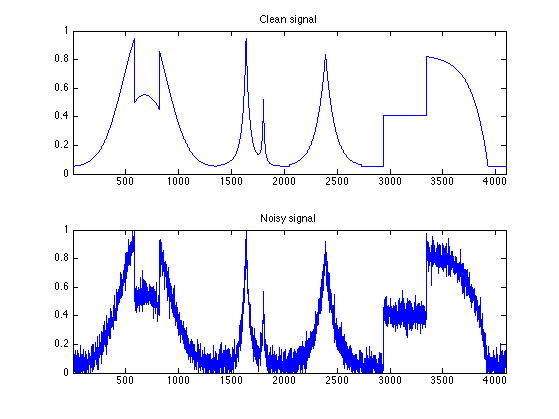## Hard Thresholding vs. Soft Thresholding

A thresholding $$\Theta : \RR^N \rightarrow \RR^N$$ is a non-linear function that operates diagonaly, i.e. $\forall i, \quad \Theta(x)_i = \th(x_i)$ where $$\th : \RR \rightarrow \RR$$ is the 1-D thresholding function.

The most important thresholding are the hard thresholding (related to $$\ell^0$$ minimization) and the soft thresholding (related to $$\ell^1$$ minimization).

The hard thresholding reads $\Theta^0_T(x)_i = \choice{ x_i \qifq \abs{x_i}>T, \\ 0 \quad \text{otherwise}. }$

Theta0 = @(x,T)x .* (abs(x)>T);


The soft thresholding reads $\Theta^1_T(x)_i = \max\pa{ 0, 1-\frac{T}{\abs{x}} } x$

Theta1 = @(x,T)max(0, 1-T./max(abs(x),1e-9)) .* x;


Display the thresholding.

t = linspace(-3,3,1024)'; T = 1;
clf;
plot( t, [Theta0(t,T), Theta1(t,T)], 'LineWidth', 2 );
axis('equal'); axis('tight');
legend('\Theta^0', '\Theta^1');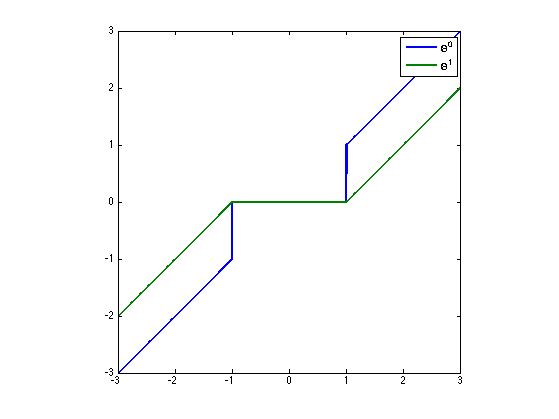## Wavelet Thresholding

It is possible to perform non linear denoising by thresholding the wavelet coefficients of $$f$$.

Shortcut for the foward orthogonal wavelet transform $$W$$ and the inverse wavelet transform $$W^{-1}=W^*$$.

options.ti = 0; Jmin = 4;
W  = @(f) perform_wavelet_transf(f,Jmin,+1,options);
Wi = @(fw)perform_wavelet_transf(fw,Jmin,-1,options);


Compute the wavelet coefficients $$x=W(f)$$.

x = W(f);


Threshold the wavelet coefficients $$\tilde x=\Theta_0(x)$$. Here we use $$T=3\si$$.

x1 = Theta0(x, 3*sigma);


Display the wavelet coefficients $$W(f)$$ and the hard thresholded coefficients $$\Theta_0(W(f))$$. Note how the wavelet coefficients are contaminated by a small amplitude Gaussian white noise, most of which are removew by thresholding.

clf;
subplot(2,1,1);
plot_wavelet(x,Jmin); axis([1 N -1 1]);
title('W(f)');
subplot(2,1,2);
plot_wavelet(Theta0(W(f),T),Jmin); axis([1 N -1 1]);
title('\Theta_0(W(f))');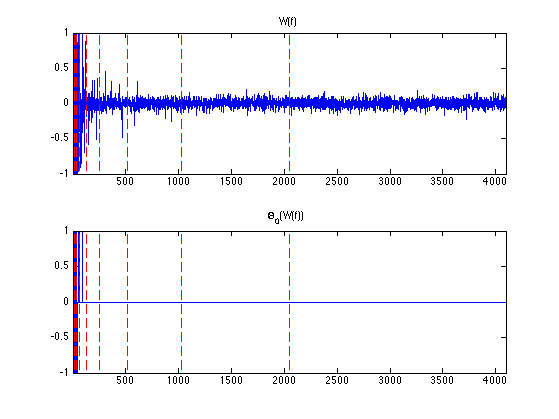Reconstruct from the thresholded coefficients the final estimator $$\tilde f = W^*(\tilde x)$$.

f1 = Wi(x1);


Display noisy and denoised signal.

clf;
subplot(2,1,1);
plot(f); axis([1 N 0 1]);
title('f');
subplot(2,1,2);
plot(f1); axis([1 N 0 1]);
title('f_1');Given a thresholding $$\Theta$$ (for instance $$\Theta^0$$ or $$\Theta^1$$), one thus defines a wavelet thresholding estimator as $\Theta_W(f) = W^* \circ \Theta \circ W.$

Operator to re-inject the coarse scale noisy coefficients. Improves a little bit the result of soft thresholding denoising (because of the bias).

x = W(f);
reinject = @(x1)assign(x1, 1:2^Jmin, x(1:2^Jmin));


Define the soft and hard thresholding estimators.

Theta0W = @(f,T)Wi(Theta0(W(f),T));
Theta1W = @(f,T)Wi(reinject(Theta1(W(f),T)));


The denoising performance of an estimator $$\tilde f = \Theta_W(f)$$ is measured using the $$L^2$$ error to the (unknown) ground trust $$f_0$$. One usually expresses it in dB using the SNR $SNR = -10\log_{10}\pa{ \frac{\norm{f_0-\tilde f}^2}{\norm{f_0}^2} }.$

Exercice 1: (check the solution) Display the evolution of the denoising SNR when $$T$$ varies. Store in fBest0 and fBest1 the optimal denoising results.

exo1;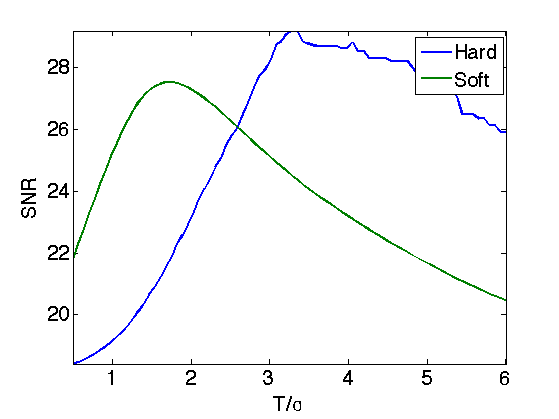Display the results. For 1-D signals, hard thresholding seems to outperform soft thresholding. For natural images, on contrary, soft thresholding seems to be better.

clf;
subplot(2,1,1);
plot(fBest0); axis([1 N 0 1]); e = snr(fBest0,f0);
title(['Hard, SNR=' num2str(e,3) 'dB']);
subplot(2,1,2);
plot(fBest1); axis([1 N 0 1]); e = snr(fBest1,f0);
title(['Soft, SNR=' num2str(e,3) 'dB']);## Translation Invariant Wavelet Transform

Orthogonal wavelet transforms are not translation invariant. It means that the processing of an image and of a translated version of the image give different results. A translation invariant wavelet transform is implemented by ommitting the sub-sampling at each stage of the transform. This correspond to the decomposition of the image in a redundant familly of $$N (J+1)$$ atoms where $$N$$ is the number of samples and $$J$$ is the number of scales of the transforms.

The foward and backward transform algorithm is the so-called "a trou" algorithm, that was introduced in [Holsch87]. See also [Fowler05] for a review. This algorithm runs in $$O(J N)$$ operations.

The invariant transform is obtained using the same function, by activating the switch options.ti=1.

options.ti = 1;
W  = @(f) perform_wavelet_transf(f,Jmin,+1,options);
Wi = @(fw)perform_wavelet_transf(fw,Jmin,-1,options);


Compute the invariant transform.

fw = W(f);


fw(:,:,1) corresponds to the low scale residual. Each fw(:,1,j) corresponds to a scale of wavelet coefficient, and has the same size as the original signal.

nJ = size(fw,3)-4;
clf;
subplot(5,1, 1);
plot(f0); axis('tight');
title('Signal');
i = 0;
for j=1:3
i = i+1;
subplot(5,1,i+1);
plot(fw(:,1,nJ-i+1)); axis('tight');
title(strcat(['Scale=' num2str(j)]));
end
subplot(5,1, 5);
plot(fw(:,1,1)); axis('tight');
title('Low scale');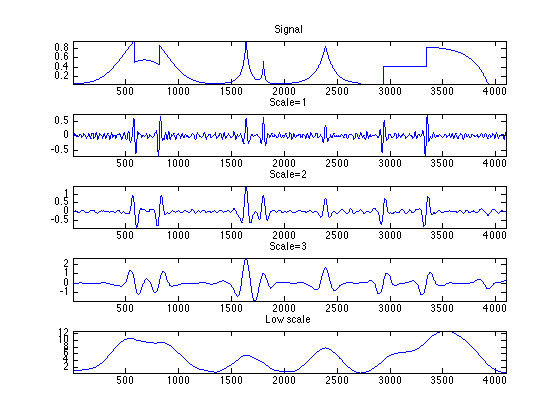## Translation Invariant Wavelet Denoising

Orthogonal wavelet denoising does not performs very well because of its lack of translation invariance. A much better result is obtained by not sub-sampling the wavelet transform, which leads to a redundant tight-frame.

Translation invariant denoising using cycle spinning is introduced in [CoifDon95]. We uwe here the a trou algorithm which is faster.

Operator to re-inject the coarse scales.

x = W(f);
reinject = @(x1)assign(x1, 1:N, x(1:N));


Define the soft and hard thresholding estimators.

Theta0W = @(f,T)Wi(Theta0(W(f),T));
Theta1W = @(f,T)Wi(reinject(Theta1(W(f),T)));


Exercice 2: (check the solution) Display the evolution of the denoising SNR when $$T$$ varies. Store in fBest0 and fBest1 the optimal denoising results.

exo2;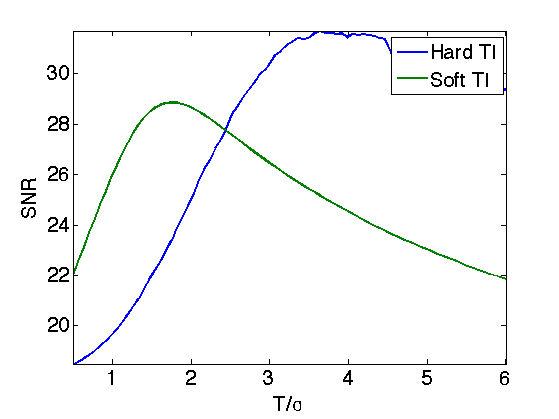Display the results.

clf;
subplot(2,1,1);
plot(fBest0); axis([1 N 0 1]); e = snr(fBest0,f0);
title(['Hard TI, SNR=' num2str(e,3) 'dB']);
subplot(2,1,2);
plot(fBest1); axis([1 N 0 1]); e = snr(fBest1,f0);
title(['Soft TI, SNR=' num2str(e,3) 'dB']);## Bibliography

• [DonJohn94] D. L. Donoho and I. M. Johnstone, Ideal spatial adaptation via wavelet shrinkage, Biometrika, vol. 81, pp. 425-455, 1994.
• [DoJoKePi95] Donoho, D.L., I.M. Johnstone, G. Kerkyacharian and D. Picard, Wavelet Shrinkage: Asymptopia, J. Roy. Statist. Soc. B 57 2, 301-369,1995.
• [CoifDon95] R.R. Coifman and D.L. Donoho, Translation-Invariant De-Noising, in Wavelets and Statistics, A. Antoniadis and G. Oppenheim, Eds. San Diego, CA: Springer-Verlag, Lecture notes 1995.
• [Fowler05] J. E. Fowler, The redundant discrete wavelet transform and additive noise, IEEE Signal Processing Letters, vol. 12, issue 9, pp. 629-632, 2005.
• [Holsch87] M. Holschneider, R. Kronland-Martinet, J. Morlet, and P. Tchamitchian, A real-time algorithm for signal analysis with the help of the wavelet transform, in Wavelets: Time-Frequency Methods and Phase Space, Springer-Verlag, 1989, pp. 286–297, 1987